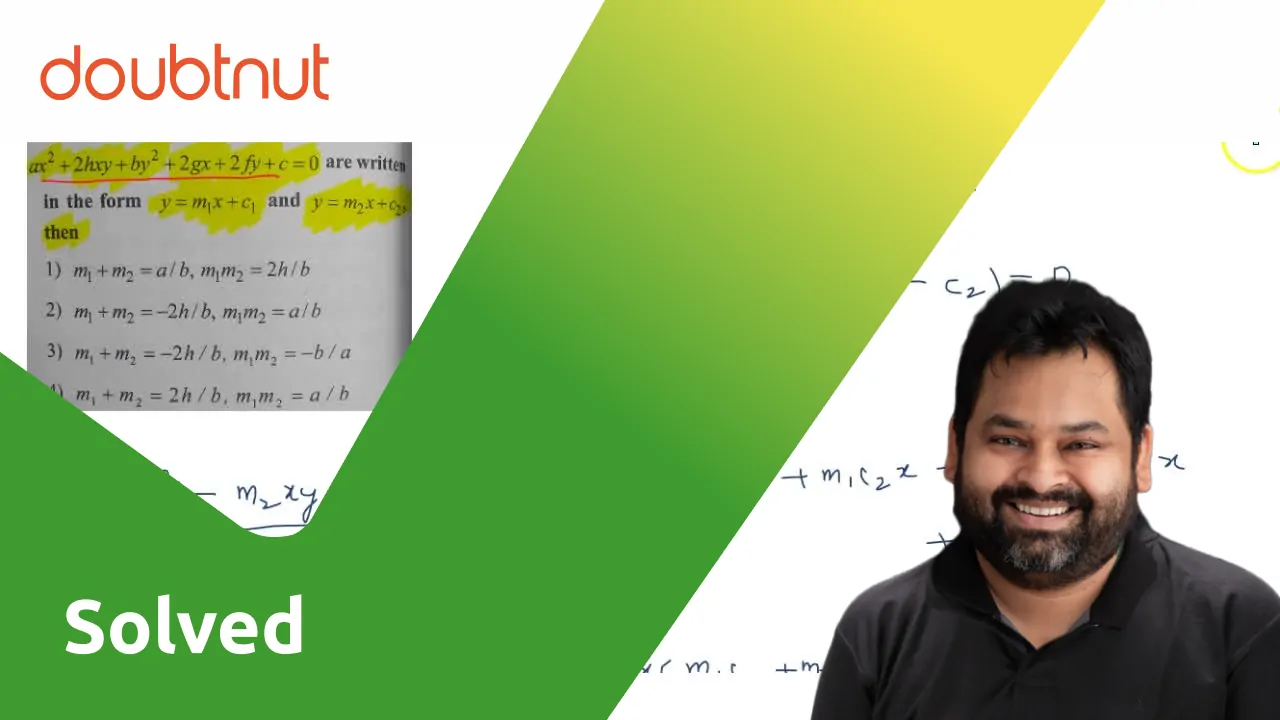Doubtnut App - Get 50 Lakh+ video solutions better than GoogleInstall Now

# 31. If the lines represented byax? + 2hxy + by2 + 2gx + 2 fy+c= 0 are writtenin the form y=mx+c1 and y = m2x+C2,then1) m + m2 = alb, mm2 = 2h1b2) m + m2 =-2h/b, mm2 = alb3) m, + m2 = -2h/b, m,m, = -b/a4) m, + m2 = 2h/b, m,m, = a / b

Video Solution